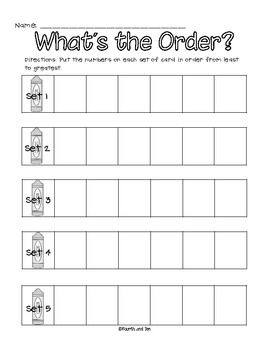Back to School Math Centers for Bigger KidsSubject
Resource Type
Product Rating
File Type

PDF (Acrobat) Document File

36 MB|53 pages
Share
Product Description
This listing is a set of beginning of the year math centers for older students. :) I am using this set of centers to review third grade skills with my fourth graders.
Included are 8 minimal prep math centers:
Sort It Out: Students solve multiplication equations and sort the products as less than 24, equal to 24, and greater than 24.
Fact Family Fun: Students review multiplication and division facts through fact families.
What's The Order: Students compare and put numbers in order from least to greatest, up to 1,000.
What's the Rule?: Students look at the beginning of a numerical pattern and determine the rule to finish the pattern.
Color the Sum: Students solve three digit addition equations (with and without regrouping) and color the even sums yellow and the odd sums orange.
Is it greater than? Is it less than? Is it equal to?: Students compare two numbers (up to the thousands) to determine whether to use the , or = symbol.
Subtraction Sort: Students sort subtraction equations by whether the problem requires regrouping or no regrouping and then solve.
Solving for N: Students solve basic algebra equations to figure out what n equals.

Recording sheets are included for all centers. :)
Total Pages
53 pages